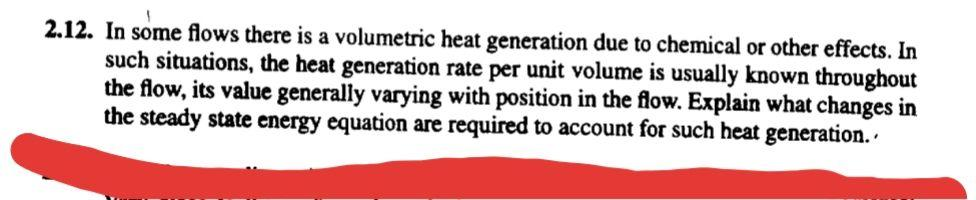# Question Solved1 Answer2.12. In some flows there is a volumetric heat generation due to chemical or other effects. In such situations, the heat generation rate per unit volume is usually known throughout the flow, its value generally varying with position in the flow. Explain what changes in the steady state energy equation are required to account for such heat generation..HSAHWR The Asker · Mechanical EngineeringTranscribed Image Text: 2.12. In some flows there is a volumetric heat generation due to chemical or other effects. In such situations, the heat generation rate per unit volume is usually known throughout the flow, its value generally varying with position in the flow. Explain what changes in the steady state energy equation are required to account for such heat generation..
More
Transcribed Image Text: 2.12. In some flows there is a volumetric heat generation due to chemical or other effects. In such situations, the heat generation rate per unit volume is usually known throughout the flow, its value generally varying with position in the flow. Explain what changes in the steady state energy equation are required to account for such heat generation..